# Antenna Basics

## Part 3 – And now for something completely different…

### Or

Well, I warned you’z people what would happen if I got no feedback regarding this series.  Today we will have a short diversion regarding decibels, which are very important in antenna theory and many other aspects of Amateur Radio!

Decibels are very important in electronics in general and Amateur Radio specifically, yet the concept is often misunderstood.  When you learn a few basic principles you will find that they are simple to work with (and they are always asked about on the Amateur Radio written qualifying exams, so it is better to be as comfortable with them as possible).

One of the first problems is that decibels are units on a “logarithmic scale”.  Most people are much more use to “linear scales”: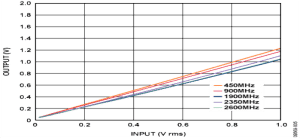You might recognize what a logarithmic scale looks like: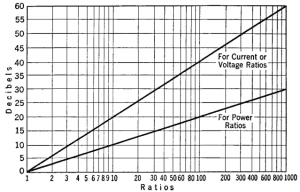This is an excellent crib sheet as well as a graphic representation to see how decibels are related to power gain and by thinking of the inverse (-dB and 1/ratios) the inverse, in power loss.

A decibel is a logarithmic unit used to express the ratio between two values of a physical quantity, often power or intensity.  It is defined as ten times the logarithm – base 10, of the ratio of two power quantities.  The decibel is one tenth of one bel – it is supposed to be named in honor of Alexander Graham Bell but is spelled “bels” not “bells” and is almost always defined in decibels, so I think Alexander got a raw deal on this honor.  The mathematical equation for a decibel is as a function of power is: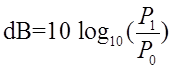Now don’t be frightened, it’s a harmless little math equation.  You can just remember a few conversion factors and you’ll never have to bother to calculate conversion factors ever again!  The simplest is 0 dB: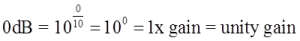(i.e. 10 watts in -> 10 watts out)

If a system has 0 dB gain it has no gain nor loss – it’s just like a 1 inch piece of wire – whatever went in goes out!10dB increase is reasonably easy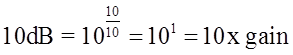(i.e. 10 watts in -> 100 watts out)

What would -10 dB of gain be or more accurately -10 dB of loss be:(i.e. 10 watts in ->> 1 watt out)

Quite simply a negative unit of decibels means you invert the ratio from x/1 to 1/x. Positive 10 dB multiplies your power by a factor of 10, MINUS 10 dB decreases your power by a factor of 1/10th!

There are a few numbers that it just pays to remember when dealing with decibels.  3 decibels is doubling the power.  When you add decibels you need to multiply their power factors, so 3 dB + 3 dB is the same as 2/1 factor increase TIMES 2/1 factor increase which equals 4/1 factor increase:

6 dB = 4x gain

If you want to show off, you can recall that adding decibels multiplies their power factor, so subtraction decibels must mean dividing their power factor:

10 dB = 10x gain    3 dB = 2x gain   10 dB -3 dB = 10x factor/2x factor   7 dB = 5x factor

Just try and remember these few numbers and by adding them together or subtracting you will figure out the power gain or loss of most situations!  Amaze and mystify your Ham friends!

Those “S” units you see on your receiver are logarithmic power scales!  One “S” unit is 6 dB each, and as we learned earlier 6 dB is an increase by a factor of 4 or a decrease by a factor of 1/4th depending on whether it is +6 dB or -6 dB.  That means if your friend is receiving you on his receiver with 6 “S” units of strength and you get a big fat linear amplifier and boost your signal sixteen times more powerful, your “booming” signal will read only a 2 “S” units increase on his S meter –  logarithmic scales can be very disappointing!

As another practical example, the other day the question was brought up regarding the power loss differences between using 40 feet of LMR 400 vs hardline transmission line for a VHF Repeater.  Well, according to one chart I looked at LMR 400 coax has 1.5 dB of loss per 100 feet of length, at VHF frequencies. Heliax hardline 1/2 inch coax has a loss of 0.814 per 100 feet.  So the difference in power loss would be:

1.5-0.814 = 0.686 dB per 100 feet or 0.686 dB/100 feet times 40feet/100feet = 0.2744 dB of additional loss using the LMR400 for the full 40 foot length.

Let’s cheat and use a calculator (I promise you the Amateur Radio Exams will all have numbers that you can do in your head)!

Power factor change = 10-0.2744/10 = .94

So, there you have it, a power loss of 6 percent!  If you remember that a single “S” unit is 6 dB of power your receiver would register how many “S” unit’s increase from this more difficult installation?

0.2744 dB change/6 dB per “S” unit = 45 THOUSANDTHS of an “S” unit!

Not exactly worth the trouble, eh?

Decibels don’t only have to be simple ratios.  You can define a specific absolute value for 0 dB and indicate that by sticking a suffix on the end of the unit.  As an example 0 dBm is 1 milliwatt of power.  So 10 dBm is equal to 10 milliwatts, 20 dBm is 100 milliwatts and 30 dBm is a watt!  There are all kinds of dB’s that use reference values in acoustics, audio electronics, radio power, antennas and many more!

Power gain/loss and antenna gain/loss are the most important kinds of dB’s for us Amateur Radio Operators.  The radiation pattern of an idealized antenna that radiates equally over 360 degrees, sorta like an incandescent light bulb uses dBi (the “I” is for isotropic).  The radiation pattern reference of a ½ λ dipole uses the term dBdAs an example, the peak gain of a dipole, perpendicular to its antenna’s axis is written as 2.15 dBi (or 1.7 times more power).  Of course, theoretically, symmetrically equally radiating antennas are and will remain theoretical – they do not exist in the real world, so using them as the standard of comparison can be considered questionable.  That is why most times papers discuss antenna gain in dBd, except when you get to marketing departments – if you use dBi you always can boast a “higher gain number in dBi units”, and that’s what they do!  So check what kind of units of gain that ad is promoting (it’s still more likely that those gain figures were not experimentally measured, or even simulated on a computer simulation, but some nice numbers that the marketing and sales departments thought would “sell”).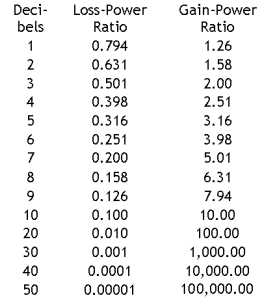Here’s a nice little crib sheet in table form, of power gain and power loss for a number of decibels.  Don’t go and memorize them, you shouldn’t need to.  This is meant as a quick reference and an opportunity to get an idea of what these numbers should mean to you.

See… that wasn’t so bad.  Now you can do all those decibel questions on the Ham license exams in your head and annoy people when you tell them how much a difference in “S” units that expensive linear amplifier is going to get them.  Have fun…

But before we leave this topic, I need to tell you about a tiny little complication that you should be aware of.  That very first equation described decibels for power.  They are a little different for different kinds of values, like voltages.  Voltages dissipate power typically proportional to the square of voltage when the impedance is held constant, so for dB of voltage ratios the equation is a little different:

So a circuit that boosts the voltage by a factor 10 has a gain of 20 dB!

Don’t worry about it, just keep it somewhere in the back of your head if you ever plan to consider ratios that aren’t direct power ratios.

Decibels are our friends.  Don’t be afraid of them.  Use them to gain a better understanding of many aspects of Amateur Radio!

Next time, just antennas, nothing but antennas (perhaps?)…

-The Editor- (I can be contacted at TheEditor@KC2RC.com)